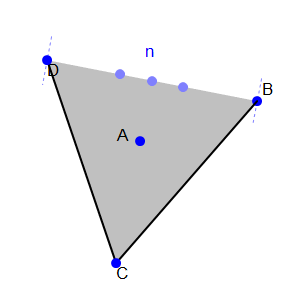# Expected Triangle In Regular N-gon

Calculus Level 1Consider the $N$ vertices of a regular $N-$gon that is inscribed in a unit circle. There are ${ N \choose 3 }$ possible subsets of 3 vertices, which form triangles. If the expected area of the triangle is

$\frac{ 5 \sqrt{ 5 + 2 \sqrt{5} } } { 24 } ,$

what is the value of $N$?

×

Problem Loading...

Note Loading...

Set Loading...